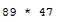## Simple Calculations in Python

You can perform calculations on numbers in Python using the four main operators:For addition, use the plus sign +

To subtract numbers, use the dash symbol  (Not an underscore _ )

For multiplication, use an asterisk * which can be made by pressing Shift and 8 on a typical keyboard.

To divide numbers, use a forward slash / (Not a backslash \ )

Type a calculation directly into a print statement to output the result.=1. Print four different simple calculations, using a different operator ( + - * / ) for each.

^ Example solution above

## Using Variables in Calculations

You can also perform calculations on variables. The example below has the values of the variables pre-written.

You need to store the result in a variable. The total variable has been used to store the result of the multiplication.=The example below has one of the variable values inputted by a user.=Don't leave the user in the dark, better user interfaces are clear and explain what outputted values mean:=1. Ask the user to input a number.

2. Divide the number by 3.

3. print the total. Don't just print the number, include "Total:"

4. Convert the total into an integer (See 3a) so it is printed as a whole number.

Example solution: